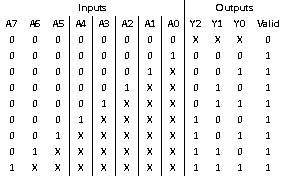Home > VHDL > Logic Circuits > 8 3 Binary Priority Encoder

8:3 Binary Priority Encoder :

The operation of the priority encoder is if two or more single bit inputs are at logic 1, then the input with the highest priority will be take importance. The coded value will be output. Table shows the truthtable of 8:3 priority.1) Using if statements :

library IEEE;

use IEEE.STD_LOGIC_1164.all;

use IEEE.NUMERIC_STD.all;

entity PRI_EN is

port (A: In unsigned (7 downto 0);

Valid: out std_logic;

Y: out unsigned (2 downto 0));

end PRI_EN;

architecture behav of PRI_EN is

begin

process (A)

begin

Valid <= '1';

If (A(7) = '1') then Y <= "111";

elsif (A(6) = '1') then Y <= "110";

elsif (A(5) = '1') then Y <= "101";

elsif (A(4) = '1') then Y <= "100";

elsif (A(3) = '1') then Y <= "011";

elsif (A(2) = '1') then Y <= "010";

elsif (A(1) = '1') then Y <= "001";

elsif (A(0) = '1') then Y <= "000";

else

Valid <= '0';

Y <= "XXX";

end if ;

end process ;

end behav;

2) Using case statements :

library IEEE;

use IEEE.STD_LOGIC_1164.all;

use IEEE.NUMERIC_STD.all;

entity PRI_EN is

port (A: In unsigned (7 downto 0);

Valid: out std_logic;

Y: out unsigned (2 downto 0));

end PRI_EN;

architecture behav of PRI_EN is

begin

process (A)

variable A_int: integer range 0 to 255;

begin

A_int := to_integer(A);

Valid <= '1';

case (A_int) is

when 128 to 255 => Y <= "111";

when 64 to 127 => Y <= "110";

when 32 to 63 => Y <= "101";

when 16 to 31 => Y <= "100";

when 8 to 15 => Y <= "011";

when 4 to 7 => Y <= "010";

when 2 to 3 => Y <= "001";

when 1 => Y <= "000";

when others => Valid <= '0';

Y <= "XXX";

end case ;

end process ;

end behav;

3) Using conditional signal assignment :

library IEEE;

use IEEE.STD_LOGIC_1164.all;

use IEEE.NUMERIC_STD.all;

entity PRI_EN is

port (A: In unsigned (7 downto 0);

Valid: out std_logic;

Y: out unsigned (2 downto 0));

end PRI_EN;

architecture behav of PRI_EN is

begin

Y<= "000" when A(0) = '1' else

"001" when A(1) = '1' else

"010" when A(2) = '1' else

"011" when A(3) = '1' else

"100" when A(4) = '1' else

"101" when A(5) = '1' else

"110" when A(6) = '1' else

"111" when A(7) = '1' else

"XXX";

end behav;

4) Using for loop statements :

A) First version :

library IEEE;

use IEEE.STD_LOGIC_1164.all;

use IEEE.NUMERIC_STD.all;

entity PRI_EN is

port (A: In unsigned (7 downto 0);

Valid: out std_logic;

Y: out unsigned (2 downto 0));

end PRI_EN;

architecture behav of PRI_EN is

begin

process (A)

begin

Valid <= '0';

Y <= "XXX";

for N in 0 to 7 loop

if (A(N) = '1') then

Y <= To_Unsigned(N, 3);

Valid <= '1';

end if ;

end loop ;

end process ;

end behav;

B) Second version :

library IEEE;

use IEEE.STD_LOGIC_1164.all;

use IEEE.NUMERIC_STD.all;

entity PRI_EN is

port (A: In unsigned (7 downto 0);

Valid: out std_logic;

Y: out unsigned (2 downto 0));

end PRI_EN;

architecture behav of PRI_EN is

begin

process (A)

begin

Valid <= '0';

Y <= "XXX";

for N in 7 downto 0 loop

if (A(N) = '1') then

Y <= To_Unsigned (N,3);

Valid <= '1';

exit ;

end if ;

end loop ;

end process ;

end behav;